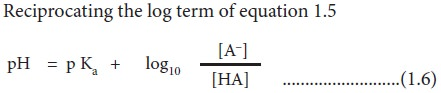Home | | BioChemistry 11th std | Henderson-Hasselbalch equation

# Henderson-Hasselbalch equation

An equation showing the relationship between a buffer’s pH and the relative amounts of the buffer’s weak acid and its conjugate base is called as Henderson-Hasselbalch equation.

Henderson–Hasselbalch equation

An equation showing the relationship between a buffer’s pH and the relative amounts of the buffer’s weak acid and its conjugate base is called as Henderson-Hasselbalch equation. Consider the dissociation of a weak acid (HA). At equilibrium,

HA ↔H+  + A

The dissociation constant (Ka) is,Reciprocating the log term of equation 1.5This form of the ionization constant equation is called the Henderson-Hasselbalch equation (equation 1.6). It is useful for calculating the pH of a weak acid solution containing its conjugate base (salt). The other forms of the Henderson-Hasselbalch equation of weak acid with its conjugate base are as follows:When the concentrations of weak acid and its conjugate base or weak base and its conjugate acid are equal, the pH of the solution equals the pKa of the buffer. This is evident from the Henderson-Hasselbalch equation.

If the pKa of bicarbonate buffer is 6.1 and the pH of blood is 7.4, then the ratio of bicarbonate to carbonic acid ([HCO3] / [H2CO3]) in blood is calculated by applying the Henderson-Hasselbalch equation.Study Material, Lecturing Notes, Assignment, Reference, Wiki description explanation, brief detail
11th Biochemistry : Chapter 1 : Basic Concepts of Bio Chemistry and Cell Biology : Henderson-Hasselbalch equation |